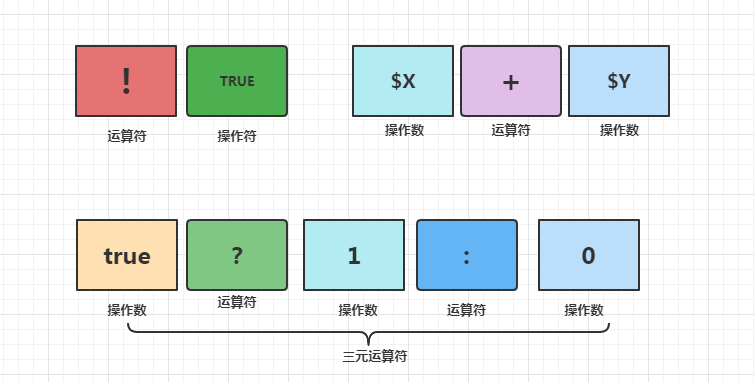# PHP字符串操作（string替换、删除、截取、复制、连接、比较、查找、包含、大小写转换、切割成数组等）

+关注继续查看

str_replace("iwind", "kiki", "i love iwind, iwind said");

str_replace(find,replace,string,count)参数 描述

find 必需。规定要查找的值。
replace 必需。规定替换 find 中的值的值。
string 必需。规定被搜索的字符串。
count 可选。一个变量，对替换数进行计数。

$string = 'fdjborsnabcdtghrjosthabcrgrjtabc';$string = preg_replace('/[abc]+/i','',$string); 方法二 把字符串转化成数组 $arr = str_split($string); foreach($arr as $key =>$value ){
if( in_array($value,array('a','b','c')) ){ unset($arr[$key]); } }$string = implode('',$arr); 三、字符串截取 <? //构造字符串$str = "ABCDEFGHIJKLMNOPQRSTUVWXYZ";
echo "原字符串：".$str." "; //按各种方式进行截取$str1 = substr($str,5); echo "从第5个字符开始取至最后：".$str1."
";
$str2 = substr($str,9,4);
echo "从第9个字符开始取4个字符：".$str2." ";$str3 = substr($str,-5); echo "取倒数5个字符：".$str3."
";
$str4 = substr($str,-8,4);
echo "从倒数第8个字符开始向后取4个字符：".$str4." ";$str5 = substr($str,-8,-2); echo "从倒数第8个字符开始取到倒数第2个字符为止：".$str5."
";
?>

/*
------------------------------------------------------

$str_cut 需要截断的字符串$length     允许字符串显示的最大长度

------------------------------------------------------
*/
function substr_cut($str_cut,$length)
{
if (strlen($str_cut) >$length)
{
for($i=0;$i < $length;$i++)
if (ord($str_cut[$i]) > 128)    $i++;$str_cut = substr($str_cut,0,$i)."..";
}
return $str_cut; } ?> 四、字符串比较 在PHP中，可以用==（双等号）或者 ===（三等号）来比较字符串。两者的区别是双等号不比较类型，三等号会比较类型，它不转换类型；用双等号进行比较时，如果等号左右两边有数字类型的值，刚会把另一个值转化为数字，然后进行比较。这样的话，如果是纯字符串或者NULL时，会转化为0进行比较。同样，大小于号也和等号一样，比较时可能出现不正确的结果。 所以，比较字符串可以用PHP的自带函数strcmp和strcasecmp。其中strcasecmp是strcmp的变种，它会先把字符串转化为小写再进行比较。 如下代码： var_dump(0 == 'Test'); var_dump(0 == ''); var_dump(5 > 'T'); var_dump(strcmp(5, 'T')); 结果为（第1～3结果是不对的，只有第4个是对的）： bool(true) bool(true) bool(true) int(-1)  五、字符串查找 strstr()函数用于获取一个指定字符串在另一个字符串中首次出现的位置到后者末尾的子字符串,如果执行成功,则返回剩余字符串(存在相匹配的字符)；如果没有找到相匹配的字符,则返回false。 语法:string strstr(string haystack,string needle) 参数:haystack:必要参数,指定从哪个字符串中进行搜索。 参数:needle:必要参数,指定搜索的对象,如果该参数是一个数值,那么将搜索与这个数值的ASCII值相匹配的字符。 实例代码如下: <?php echo strstr(“明日编程词典”,”编”); //输出查询的字符串 echo “<br>”; //执行回车 echo strstr(“www.phpfensi.com”,”111”); //输出查询的字符串（从第一个m取值） echo “<br>”; //执行回车 echo strstr(“0431-84972266″,”8″); //输出查询的字符串 ?>  定义和用法:strpos() 函数返回字符串在另一个字符串中第一次出现的位置,如果没有找到该字符串,则返回 false。 语法:strpos(string,find,start) 参数:string 必需,规定被搜索的字符串。 参数:find 必需,规定要查找的字符。 参数:start 可选,规定开始搜索的位置。 注释：该函数对大小写敏感,如需进行对大小写不敏感的搜索,请使用 stripos() 函数。 实例代码如下: <?php echo strpos("Hello world!","wo"); ?>//输出：6  六、字符串大小写转换 <?php$foo = 'hello world!';
$foo = ucwords($foo); // Hello World!$bar = 'HELLO WORLD!';$bar = ucwords($bar); // HELLO WORLD!$bar = ucwords(strtolower($bar)); // Hello World! ?> 第一个词首字母变大写：ucfirst() <?php$foo = 'hello world!';
$foo = ucfirst($foo); // Hello world!$bar = 'HELLO WORLD!';$bar = ucfirst($bar); // HELLO WORLD!$bar = ucfirst(strtolower($bar)); // Hello world! ?> 第一个词首字母小写lcfirst() <?php$foo = 'HelloWorld';
$foo = lcfirst($foo); // helloWorld
$bar = 'HELLO WORLD!';$bar = lcfirst($bar); // hELLO WORLD!$bar = lcfirst(strtoupper($bar)); // hELLO WORLD! ?> 字母变大写：strtoupper() 字母变小写：strtolower() 七、字符串切割 <?php$str="1|2|3|4|5|";
$var=explode("|",$str);
print_r($var); ?>$var=explode("|",$str); 把$str按|进行分割，php还有其他的把字符串指定字符分割成数组

str_split(string,length)参数 描述
string 必需。规定要分割的字符串。
length 可选。规定每个数组元素的长度。默认是 1。
json_decode()这个函数也可以把字符串分割成数组 （第二个参数为true才行）PHP去掉名称字符串中的表情
PHP去掉名称字符串中的表情
13 0PHP/Python/js/Golang/Java：时间转为人类可读的字符串格式：刚刚、几分钟前、几小时前、几天前
PHP/Python/js/Golang/Java：时间转为人类可读的字符串格式：刚刚、几分钟前、几小时前、几天前
25 0PHP实现截取中文字符串不出现?号的解决方法
PHP实现截取中文字符串不出现?号的解决方法
23 0PHP为什么需要字符串运算？底层原理是什么？
PHP为什么需要字符串运算？底层原理是什么？
31 0PHP中的字符串有长度大小限制吗?为什么？
PHP中的字符串有长度大小限制吗?为什么？
60 0PHP中的字符串是干什么的？底层原理是什么？
PHP中的字符串是干什么的？底层原理是什么？
49 0PHP官方手册研读--数据类型：字符串

54 0【web开发基础】PHP快速入门（5）-PHP运算符之算术运算符和字符串运算符详解

78 0php levenshtein,php字符串编辑距离
php levenshtein,php字符串编辑距离
55 0PHP 零基础入门笔记（11）：字符串 String
PHP 零基础入门笔记（11）：字符串 String
58 0

PHP完全自学手册文档教程946088

PHP 2017.北京 全球开发者大会——高可用的PHP